Homework Help Question & Answers

# Consider the differential equation 4x2y′′ − 8x2y′ + (4x2 + 1)y = 0 (a) Verify that x0 = 0 is a regular singular point of...

Consider the differential equation

4x2y′′ − 8x2y′ + (4x2 + 1)y = 0

(a) Verify that x0 = 0 is a regular singular point of the differential equation and then find one solution as a Frobenius series centered at x0 = 0. The indicial equation has a single root with multiplicity two. Therefore the differential equation has only one Frobenius series solution. Write your solution in terms of familiar elementary functions.

(b) Use Reduction of Order to find a second linearly independent solution and then form the general solution of the differential equation.

Note: In part (a), you will find one solution of the differential equation is y = √xex. You must show all of the work of finding the series in part (a), but if you cannot, proceed to reduction of order with the given function.

#### Homework Answers

Answer #1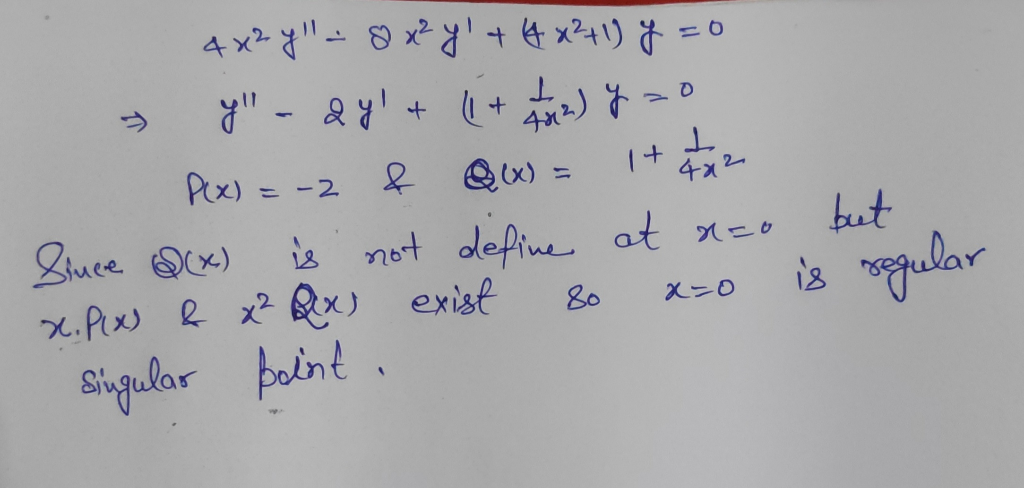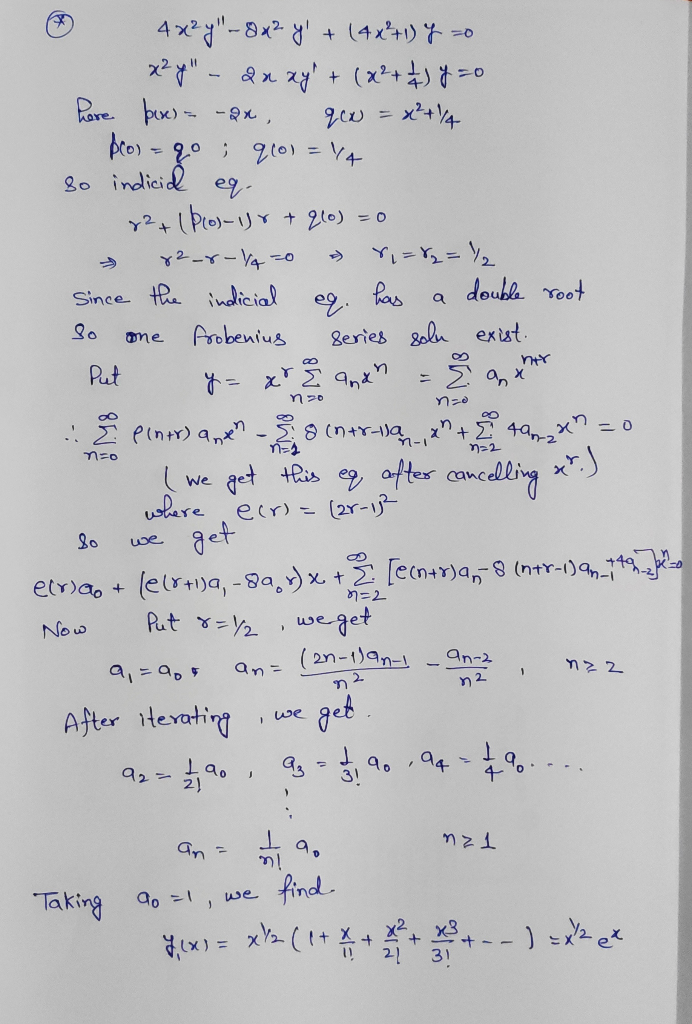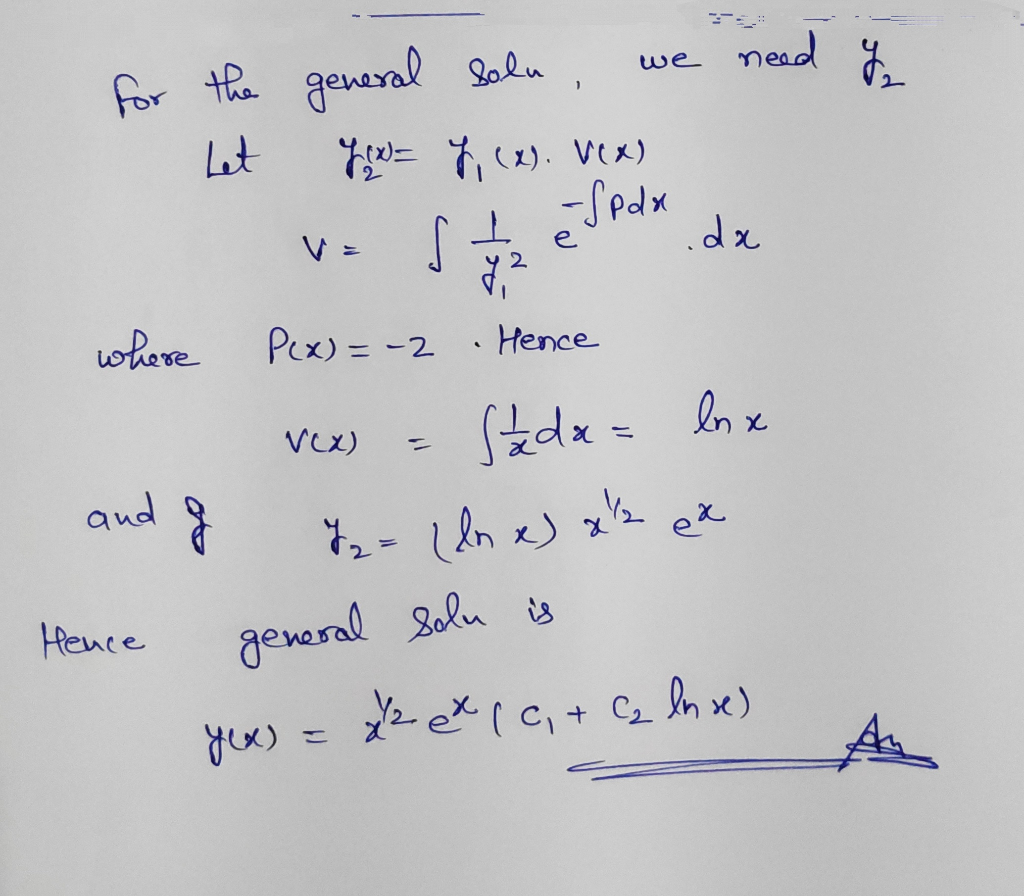Know the answer?
Your Answer:

#### Post as a guest

Your Name:

What's your source?

#### Earn Coin

Coins can be redeemed for fabulous gifts.

Not the answer you're looking for? Ask your own homework help question. Our experts will answer your question WITHIN MINUTES for Free.
Similar Homework Help Questions
• ### 20. 0/2 points | Previous Answers ZillDiffEQ9 6.3.018 The point x 0 is a regular singular point o...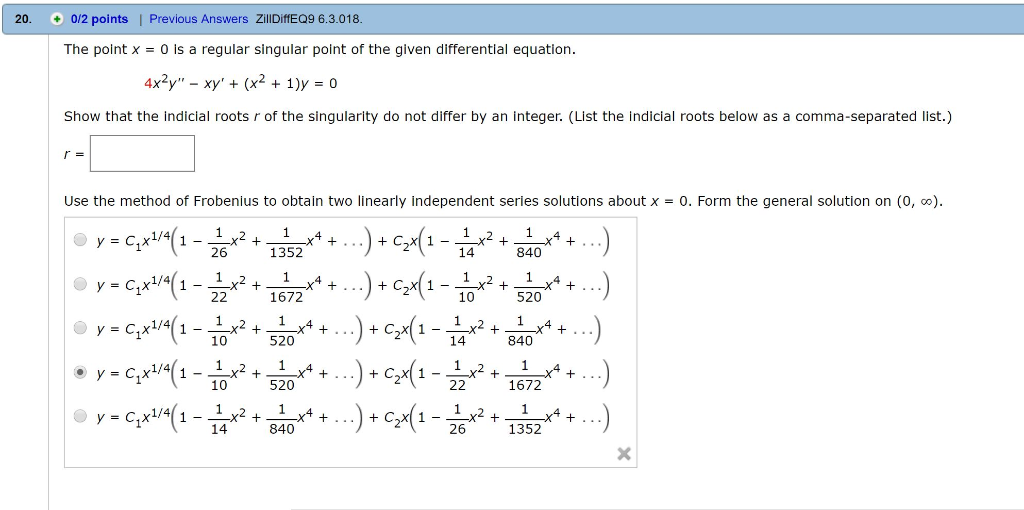20. 0/2 points | Previous Answers ZillDiffEQ9 6.3.018 The point x 0 is a regular singular point of the given differential equation. 4x2y"-xy + (x2 + 1)y = 0 Show that the indicial roots r of the singularity do not differ by an integer. (List the indicial roots below as a comma-separated list.) Use the method of Frobenius to obtain two linearly independent series solutions about x-0. Form the general solution on (0, ) 2015 340**. y = C-X1/4 1672...

• ### Given that x =0 is a regular singular point of the given differential equation, show that...

Given that x =0 is a regular singular point of the given differential equation, show that the indicial roots of the singularity do not differ by an integer. Use the method of Frobenius to obtain two linearly independent series solutions about x = 0. Form the general solution on (0, ∞) 2xy''-y'+y=0

• ### 4. Given that x =0 is a regular singular point of the given differential equation, show...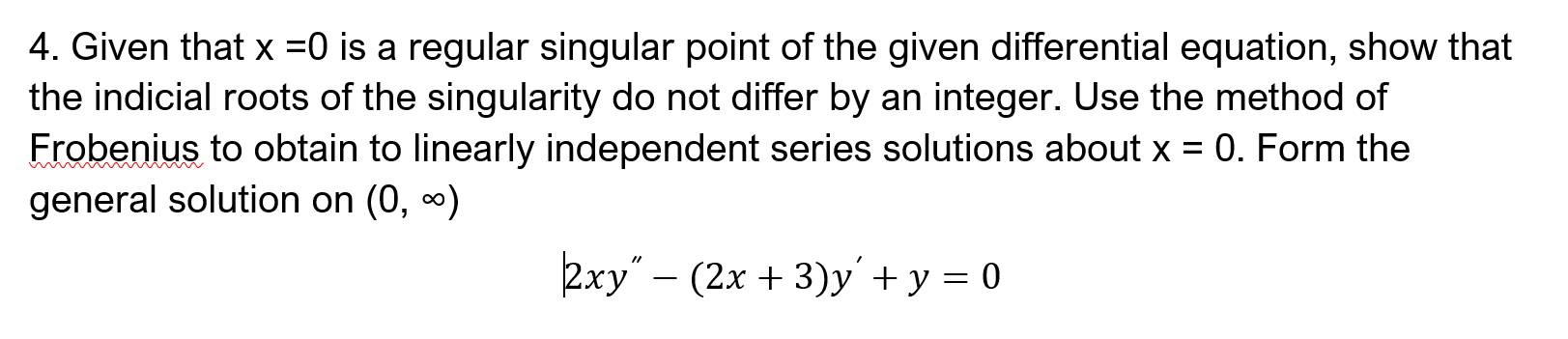4. Given that x =0 is a regular singular point of the given differential equation, show that the indicial roots of the singularity do not differ by an integer. Use the method of Frobenius to obtain to linearly independent series solutions about x = 0. Form the general solution on (0, 0) kxy” – (2x + 3)y' + y = 0

• ### 1) Show that zo-0 is a regular singular point for the diferenta equation Zo = 0 is a regular sing...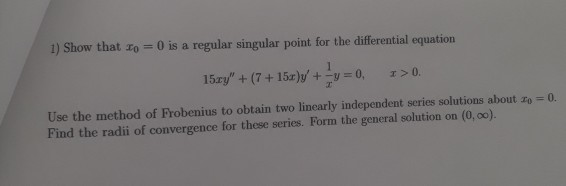please show the recurrence formula 1) Show that zo-0 is a regular singular point for the diferenta equation Zo = 0 is a regular singular point for the differential equation 15ェy" + (7 + 15r)y, +-y = 0, x>0. Use the method of Frobenius to obtain two linearly independent series solutions about zo Find the radii of convergence for these series. Form the general solution on (0, 0o). 0. 1) Show that zo-0 is a regular singular point for the...

• ### 1 point) Consider the differential equation which has a regular singular point at x = O. The indi...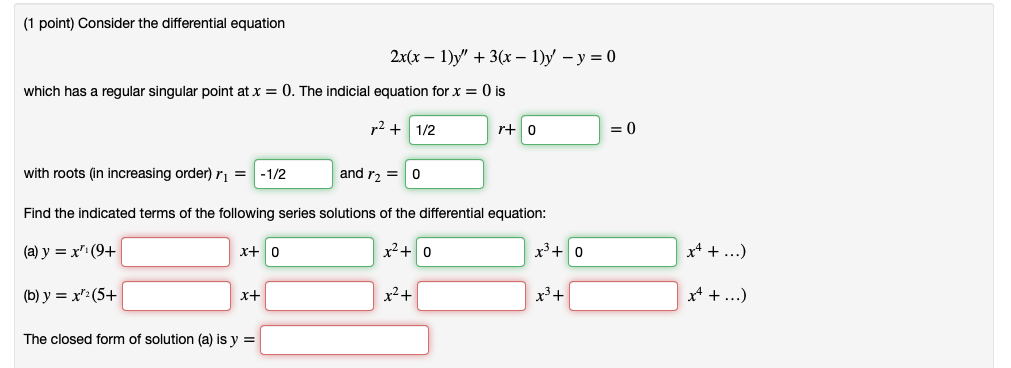1 point) Consider the differential equation which has a regular singular point at x = O. The indicial equation for x 0 is r1/2 r+ 0 =0 and r O with roots (in increasing order) r1/2 Find the indicated terms of the following series solutions of the differential equation: (a) y = x, (94 (b)y-x(5+ The closed form of solution (a) is y = xtO r3+ 1 point) Consider the differential equation which has a regular singular point at x...

• ### (1 point) Consider the differential equation 2x(x )y"3 - 1)y -y0 which has a regular singular...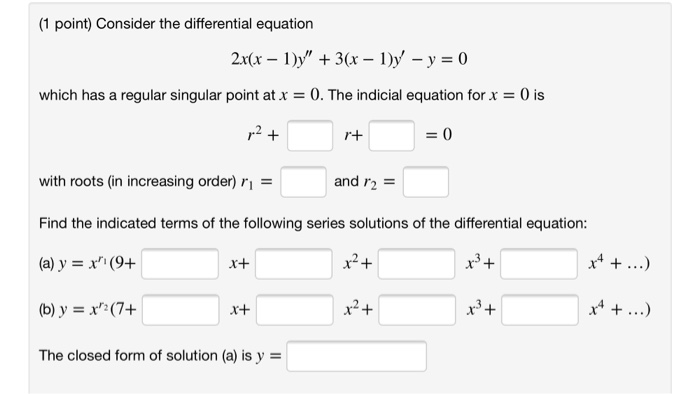(1 point) Consider the differential equation 2x(x )y"3 - 1)y -y0 which has a regular singular point atx 0. The indicial equation for x 0 is 2+ 0 r+ with roots (in increasing order) r and r2 Find the indicated terms of the following series solutions of the differential equation: x4. (a) y =x (9+ x+ (b) y x(7+ The closed form of solution (a) is y (1 point) Consider the differential equation 2x(x )y"3 - 1)y -y0 which has...

• ### Engineering Mathematics IIA Page 3 of 8 3. Consider the second-order ordinary differential equation for y(x)...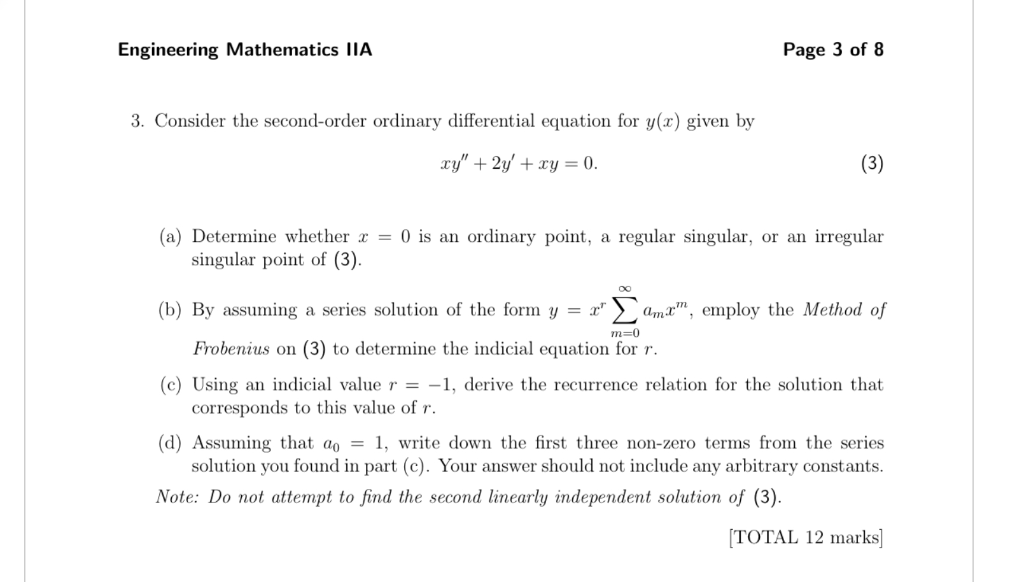Engineering Mathematics IIA Page 3 of 8 3. Consider the second-order ordinary differential equation for y(x) given by (3) xy"2y' +xy = 0. (a) Determine whether = 0 is an ordinary point, regular singular, or an irregular a singular point of (3). (b) By assuming a series solution of the form y = x ama, employ the Method of m-0 Frobenius on (3) to determine the indicial equation for r. (c) Using an indicial value r = -1, derive the...

• ### In each of Problems 1 through 6: a. Show that the given differential equation has a regular singu...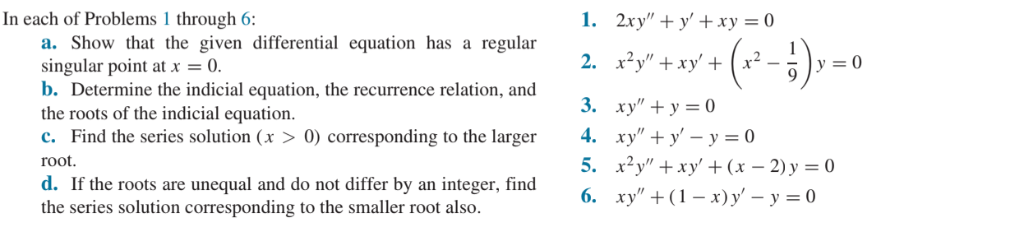Do JUST # 2 please In each of Problems 1 through 6: a. Show that the given differential equation has a regular singular point at x0. b. Determine the indicial equation, the recurrence relation, and the roots of the indicial equation. c. Find the series solution (x >0) corresponding to the larger root. d. If the roots are unequal and do not differ by an integer, find the series solution corresponding to the smaller root also. 2. xy" +xy+ 3....

• ### In each of Problems 1 through 6: a. Show that the given differential equation has a regular singu...Do JUST # 3 Please In each of Problems 1 through 6: a. Show that the given differential equation has a regular singular point at x0. b. Determine the indicial equation, the recurrence relation, and the roots of the indicial equation. c. Find the series solution (x >0) corresponding to the larger root. d. If the roots are unequal and do not differ by an integer, find the series solution corresponding to the smaller root also. 2. xy" +xy+ 3....

• ### (1 point) In this problem you will solve the differential equation or @() (1) Since P(a) 0 are not analytic at and 2()...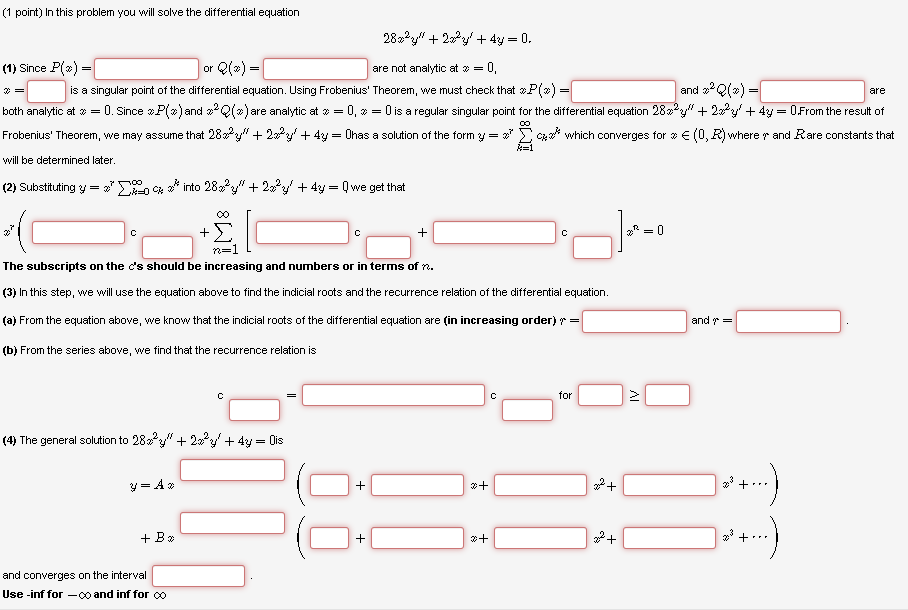(1 point) In this problem you will solve the differential equation or @() (1) Since P(a) 0 are not analytic at and 2() is a singular point of the differential equation. Using Frobenius' Theorem, we must check that are both analytic a # 0. Since #P 2 and #2e(z) are analytic a # 0-0 is a regular singular point for the differential equation 28x2y® + 22,23, + 4y 0 From the result ol Frobenius Theorem, we may assume that 2822y"...

Free Homework App

Scan Your Homework
to Get Instant Free Answers
Need Online Homework Help?

Get Answers For Free
Most questions answered within 3 hours.